# Cryptography: A Closer Look at the Algorithms

### 要約

This tutorial is a part of our series on cryptography, designed to help the product design engineer quickly gain an understanding of how the technique helps protect designs. This installment discusses how modern cryptographic algorithms are implemented. A similar version of this application note was originally published on April 30, 2020 on Electronic Design.

### Introduction

In the last two installments in our cryptography series of tutorials, we covered the basic concepts and the two basic types of cryptography. This segment discusses specific implementation details of the most common cryptographic algorithms, starting with the fundamental XOR function and then moving into more complex symmetric and asymmetric algorithms in use today. This piece concludes with a review of how an asymmetric key algorithm can be used to exchange a shared private key. This enables the use of faster symmetric key algorithms to exchange bulk-encrypted data without developing elaborate key exchange systems.

### XOR Function: A Vital Logical Operation

XOR (exclusive or) is a vital logical operation that is used in various capacities in many, if not all, cryptographic algorithms. Figure 1 shows how this function works. You’ll want to understand this function before diving into the algorithms.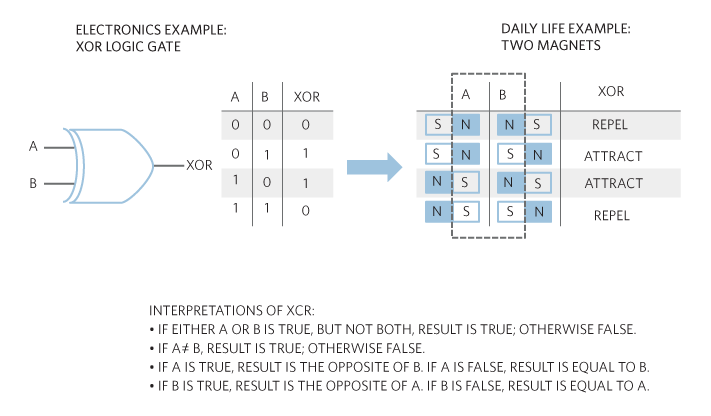### Exclusive OR (XOR) – A Fundamental Element of Reversible (i.e. Lossless) Encryption

Because of the properties of XOR, one of the inputs can be used as a key for data going into the other input. For instance, if A is a single bit of an encryption key, an XOR with a data bit from B flips the bit if A is a 1. This can be reversed by bitwise XOR’ing the encrypted result with the key again.

Let’s look at an example:

Our goal is to take the word “Secret,” encrypt it with a key using XOR, then decrypt it using the same key and the XOR function. These are the steps:

1. Choose a key. We will choose the letter “k” as our key.
2. Convert the letter “k” to binary using the ASCII (American Standard Code for Information Interchange) character encoding standard. The result is: 01101011
3. Convert the word “Secret” to binary. The result is: 01010011 01100101 01100011 01110010 01100101 01110100
4. XOR each letter in “Secret” with “k” the key letter. This gives us the encrypted value.

 S e c r e t 01010011 01100101 01100011 01110010 01100101 01110100
XOR the “key”: 01101011 01101011 01101011 01101011 01101011 01101011
Encrypted Value: 00111000 00001110 00001000 00011001 00001110 00011111
5. Now to decrypt the encrypted value, we XOR it with the key letter “k.” This step gives us back our original “Secret” word.

Encrypted Value: 00111000 00001110 00001000 00011001 00001110 00011111
XOR the “Key”: 01101011 01101011 01101011 01101011 01101011 01101011

 Decrypted Value: 01010011 01100101 01100011 01110010 01100101 01110100 S e c r e t

### SHA (Secure Hash Algorithm)

basic idea behind a SHA function is to take data of a variable size and condense it into a fixed-size bit-string output. This concept is called hashing. The SHA functions are a family of hashing algorithms that have been developed over time through NIST (National Institute of Standards and Technology) oversight. The SHA-3 function is the latest.

Figure 2 shows the basic concept of secure hash generation.The SHA function has the following characteristics:

1. Variable input length.
2. Fixed output length.
3. One-way function. In Figure 2, it is impossible to use the resultant hash value to regenerate the input text, other than trying each possible input text. This becomes computationally impossible for sufficiently large inputs.
4. If the same input message is fed to the SHA function, it will always generate the same resultant hash.
5. It is not possible to generate the same hash value using two different input values. This is called "Collision Resistance."
6. A small change in the input value, even a single bit, completely changes the resultant hash value. This is called the "Avalanche Effect."

If a hash function satisfies all the above, it is considered a strong hash function.

Some of the SHA functions currently in use are:

• SHA-1
• SHA-2
• SHA-3

Now let's explore how SHA functions work, with a focus on SHA-2 and SHA-3. SHA-1 is being phased out and is not recommended for any new designs.

### The Ins and Outs of SHA-2

The SHA-2 function has four main types based on output bit length:

1. SHA-224 – hash is 224 bits long.
2. SHA-256 – hash is 256 bits long.
3. SHA-384 – hash is 384 bits long.
4. SHA-512 – hash is 512 bits long.

Let's look at SHA-256 as an example. Figure 3 shows a block diagram of a SHA-256 engine.### Secure Hash Generation: SHA-256 Function

The input message is first padded to make sure that it will completely fit in “n” number of 512-bit blocks. The first 512-bit block is then fed into a compression function along with an initial 256-bit hash value. The compression function essentially shuffles the message 64 times before compressing it to 256 bits and sending it out to the next compression block or sending it out as the final hash. Thus, a variable input message gets shuffled many times to prevent it from being used to get to the original message. Once that is done, the output hash is generated.

### How SHA-3 Works

The SHA-3 function has no predefined output length. The input and output lengths have no maximums either. But for comparison purposes with SHA-2, let’s define four main types based on output bit lengths. These are:

1. SHA3-224 – hash is 224 bits long.
2. SHA3-256 – hash is 256 bits long.
3. SHA3-384 – hash is 384 bits long.
4. SHA3-512 – hash is 512 bits long.

Let’s look at SHA3-256 as an example. SHA-3 uses a Keccak sponge function. Just like a sponge, the first step soaks in or absorbs the input message. In the next phase, the output hash is squeezed out. Figure 4 is a block diagram of a SHA3-256 function.### Secure Hash Generation: SHA3-256 Function

The iteration function in Figure 4 takes in the 1600 bits of data and then puts it through 24 rounds of permutation using a specific algorithm and then passes it to the next stage as a 1600-bit block. This continues until the absorbing phase has completed.

Once the absorbing phase has been completed, the last 1600-bit block is passed to the squeezing phase. In this case, since the SHA3-256 output hash length is less than 1088 bits, the squeezing phase does not need any iteration functions. We take the first 256 bits from the last stage and that is the output hash.

For example, if the required hash length was 2500 bits, we would have needed three more instances of the iteration function to get the desired length hash.

Like older encryption algorithms such as DES (Data Encryption Standard) and 3DES (Triple Data Encryption Standard), the purpose of the AES algorithm is to scramble and substitute input data based on the value of an input key in a reversible way. The result is called Ciphertext. The AES algorithm was designed to replace the DES and 3DES algorithms developed in prior decades and which are vulnerable to attack. A description of the AES algorithm is shown in Figure 5.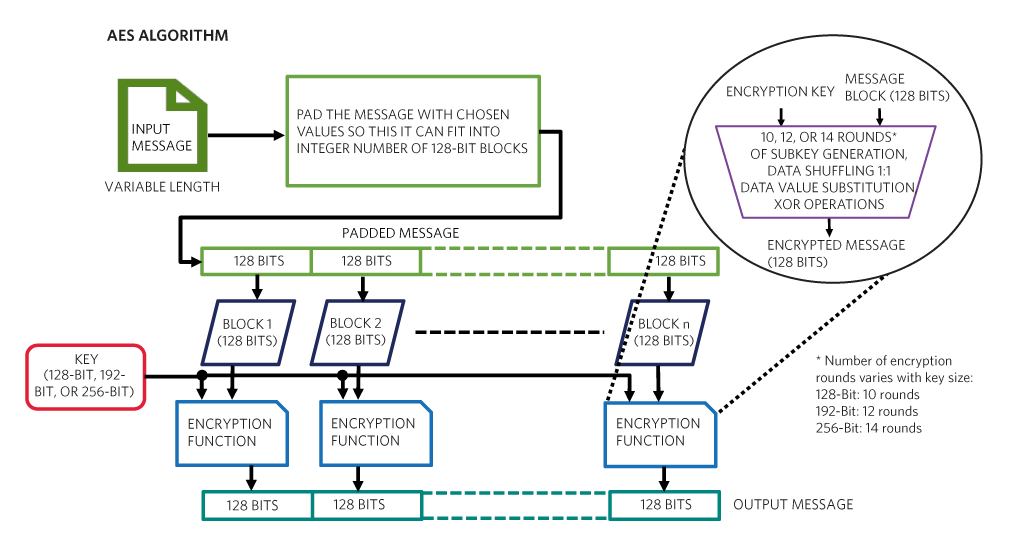### AES Algorithm

The AES algorithm is a fixed-width encryption algorithm. Therefore, the input message is first padded to make sure that it will completely fit in “n” number of 128-bit blocks.

Each 128-bit block is fed into the encryption algorithm along with an encryption key. Depending on the number of bits in the encryption key, the AES algorithm performs a certain number of rounds of obscuring the input block bits. This obscuring is accomplished by shuffling data bits, taking portions of the data and substituting them with values from a look-up table (like a decoder wheel), and performing XOR operations to flip bits from 0 to 1 according to bit values in a set of “round keys” generated from the input encryption key. A round key is used one time for one of the obscuring rounds and is created by “expanding” a portion of the encryption key by copying bits and inserting the copies in-between other bits.

The AES decryption function simply performs the reverse of the operations in the encryption function using the same encryption key in order to unscramble the original input block data.

### 3DES (Triple Data Encryption Standard)

The basic idea behind the Triple DES (or 3DES) algorithm is to scramble and substitute input data based on the value of an input key in a reversible way. The result is called ciphertext. The 3DES algorithm is a reprise of the original DES (Data Encryption Standard) algorithm developed in the 1970s. When DES was compromised in the 1990s, the need for a more secure algorithm was clear. 3DES became the near-term solution to the problems with single DES. In order to understand 3DES, a description of the original DES is first shown in Figure 6.### DES (Data Encryption Standard) Algorithm

The DES algorithm is a fixed-width encryption algorithm. Therefore, the input message is first padded to make sure that it will completely fit in “n” number of 64-bit blocks.

Each 64-bit block is fed into the encryption algorithm along with a 56-bit encryption key (most versions of the algorithm take a 64-bit key, but 8 bits are ignored). The encryption function uses the input key to generate 16 “subkeys,” each used for 16 rounds of obscuring the input block bits. This obscuring is accomplished by shuffling data bits, taking portions of the data and substituting them with values from a lookup table (like a decoder wheel), and performing XOR operations to flip bits from 0 to 1 according to the values of bits in the subkeys.

The DES decryption function simply performs the reverse of the operations in the encryption function using the same encryption key to unscramble the original input block data.

How does 3DES work?

### Deriving the Triple DES Algorithm from DES

After DES was shown to be vulnerable to attacks shorter than a “brute-force attack” (cycling through every possible key value until the original message blocks are revealed), a simple method of effectively increasing the size of the encryption key was developed. Figure 7 depicts the 3DES solution.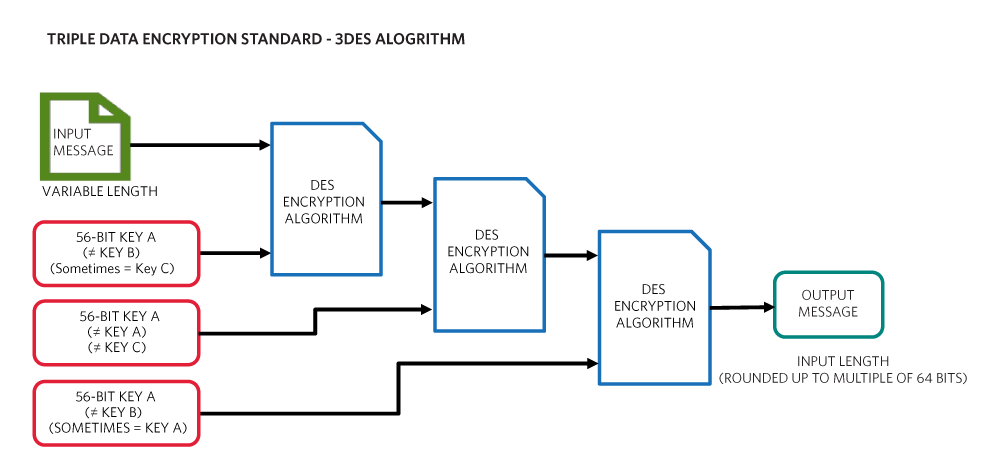The 3DES algorithm is literally 3 DES operations. The first and last operations are encryption operations, while the middle operation is a decryption operation. It is important to note that “encryption” and “decryption” are just names assigned to scrambling operations that are the reverse of each other.

For each of the DES operations performed in 3DES, a dedicated key is used for that operation. Often, the key for the first and third operations is the same. Using the same key for the first and third operations and using a different key for the middle operation effectively doubles the total key length, which makes a brute force attack much harder and eliminates the vulnerabilities of a single DES.

### RSA Public Key Cryptosystem

RSA is named after its creators, Ron Rivest, Adi Shamir, and Leonard Adleman, and is one of the first asymmetric public-key encryption/decryption systems. It uses the properties of modular arithmetic of prime numbers to generate a public key that can be used for encryption and a private key that can be used for decryption. The encryption and decryption operations are also based in modular arithmetic. An overview of RSA is shown in Figure 8.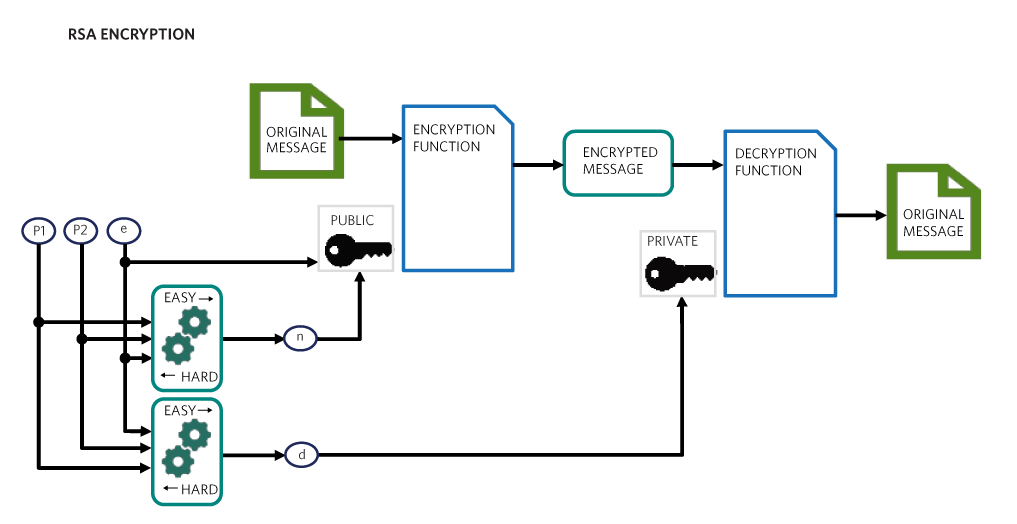The key generation and encryption/decryption operations are known as 1-way or “trapdoor” functions. They are mathematical operations that are relatively simple to calculate in one direction, but difficult to calculate in the other direction. For instance, it is easy to calculate times 2, but harder to calculate the square root of x.

In the case of RSA, two large prime numbers are multiplied together to create a part of the public and private keys. The multiplication is easy; factoring back to discover the secret prime numbers is difficult.

It is also much easier to encrypt a message with the public key than to try to go in reverse to obtain the message without the private key. However, the private key can also easily unlock the message, and must therefore never be shared.

The private key can be viewed as opening a trapdoor, revealing a shortcut to bypass the complex maze of attempting to breaking an encrypted message.

RSA security relies on large prime numbers and complex operations. Even the easy path through its trapdoor functions with large keys is cumbersome for most computing systems. Therefore, RSA is often used as a vehicle to send shared encryption keys that can be used in faster, symmetrical algorithms like DES, 3DES, and AES for individual transactions.

### ECDSA Algorithm

The Elliptic Curve Digital Signature Algorithm (ECDSA) allows a participant in a communication to prove authenticity by generating a digital signature for an input message based on a hidden piece of information known as a private key. This key is used to generate a public key that is used by others to verify the participant's authenticity.

Digital signatures are generated with an input message, a private key, and a random number. The public key can then be used to verify that the signer (or participant) is in possession of the corresponding private key and is therefore authentic. This concept is illustrated in Figure 9.The digital signature algorithm was first introduced with modular arithmetic, which depends on large prime numbers and calculations that require heavy use of computing power. The introduction of elliptic curve cryptography utilizes the mathematical properties of elliptic functions to simplify the math without sacrificing security.

The key generation and signing operations are known as 1-way or “trapdoor” functions. They are elliptic curve calculations that are relatively simple to compute in one direction, but difficult to compute in the other direction. The private key can be viewed as opening a trapdoor, revealing a shortcut to bypass the complex maze of attempts to break a key generation or signing operation.

ECDSA allows one party to sign messages from any party. However, to prove authenticity with ECDSA, a signer must not have foreknowledge of the message to be signed. This lack of control over the message allows another participant in communication to “challenge” the signer with new information to prove possession of the private key.

### ECDH Key Exchange Protocol

The Elliptic Curve Diffie-Hellman (ECDH) key exchange allows for two parties to establish a shared key for communication with only one piece of hidden information called a private key. Without the private key of one of the parties involved, an eavesdropper cannot easily determine the shared key. However, the algorithm allows the private key of one party and the public key of the other party to be combined to produce a resulting key that is the same for both parties. This concept is illustrated in Figure 10.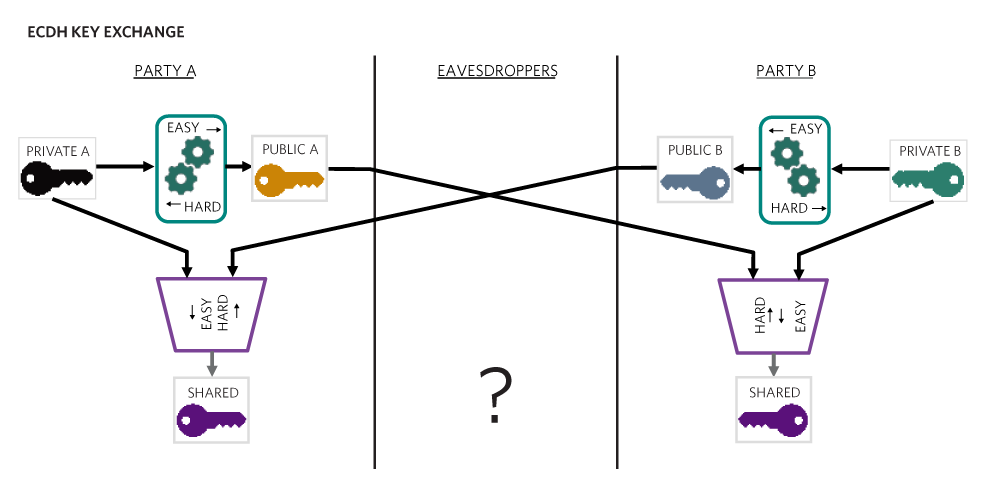### ECDH Key Exchange

The Diffie-Hellman key exchange was first introduced with modular arithmetic, which depends on large prime numbers and calculations that require heavy use of computing power. The introduction of elliptic curve cryptography utilizes the mathematical properties of elliptic functions to simplify the math without sacrificing security.

The key generation and key combination operations are known as 1-way or “trapdoor” functions. They are elliptic curve calculations that are relatively simple to compute in one direction, but difficult to compute in the other direction. The private key can be viewed as opening a trapdoor, revealing a shortcut to bypass the complex maze of attempts to break a key generation or combination operation.

The ECDH algorithm allows two parties to establish a key together, but it does not guarantee that either party is to be trusted. For this, additional layers of authentication are required. For instance, if a public key is given a certificate such as an ECDSA (Elliptic Curve Digital Signature Algorithm) signature calculated with a private key from a trusted key holder, the certification of the public key is verified by authenticating the certificate with the trusted holder’s public key.

By using public keys with certificates from a trusted authority, participants in ECDH can be certain that their counterpart is an authentic participant. Watch for other segments in our series of cryptography tutorials to continue deepening your understanding of this important security technique.